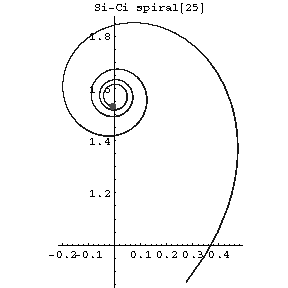# Si-ci-spiral

Jump to: navigation, search

A plane curve whose equation in rectangular Cartesian coordinates $(x,y)$ has the form

$$x=\operatorname{ci}(t),\quad y=\operatorname{si}(t),$$

where $\operatorname{ci}$ is the integral cosine, $\operatorname{si}$ is the integral sine and $t$ is a real parameter (see Fig.).Figure: s084980a

The arc length from $t=0$ to $t=t_0$ is equal to $\log t_0$, and the curvature is equal to $\kappa=t_0$.